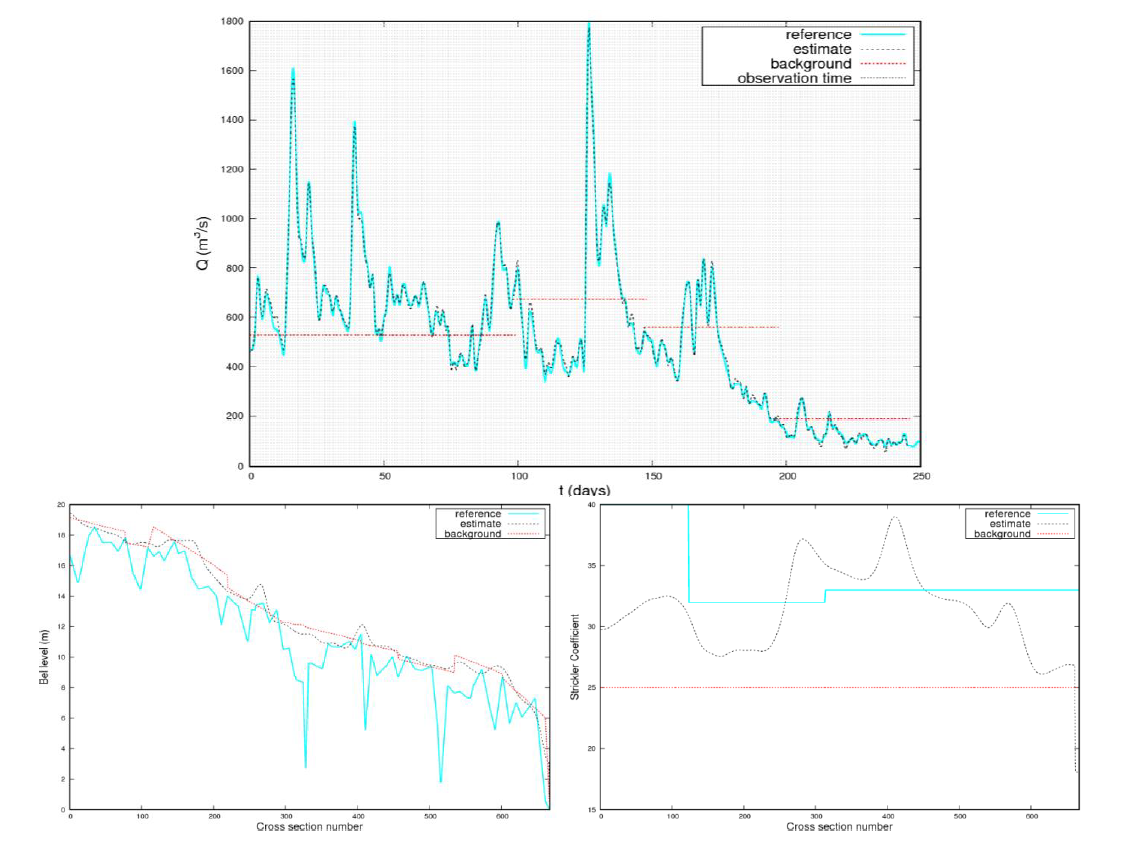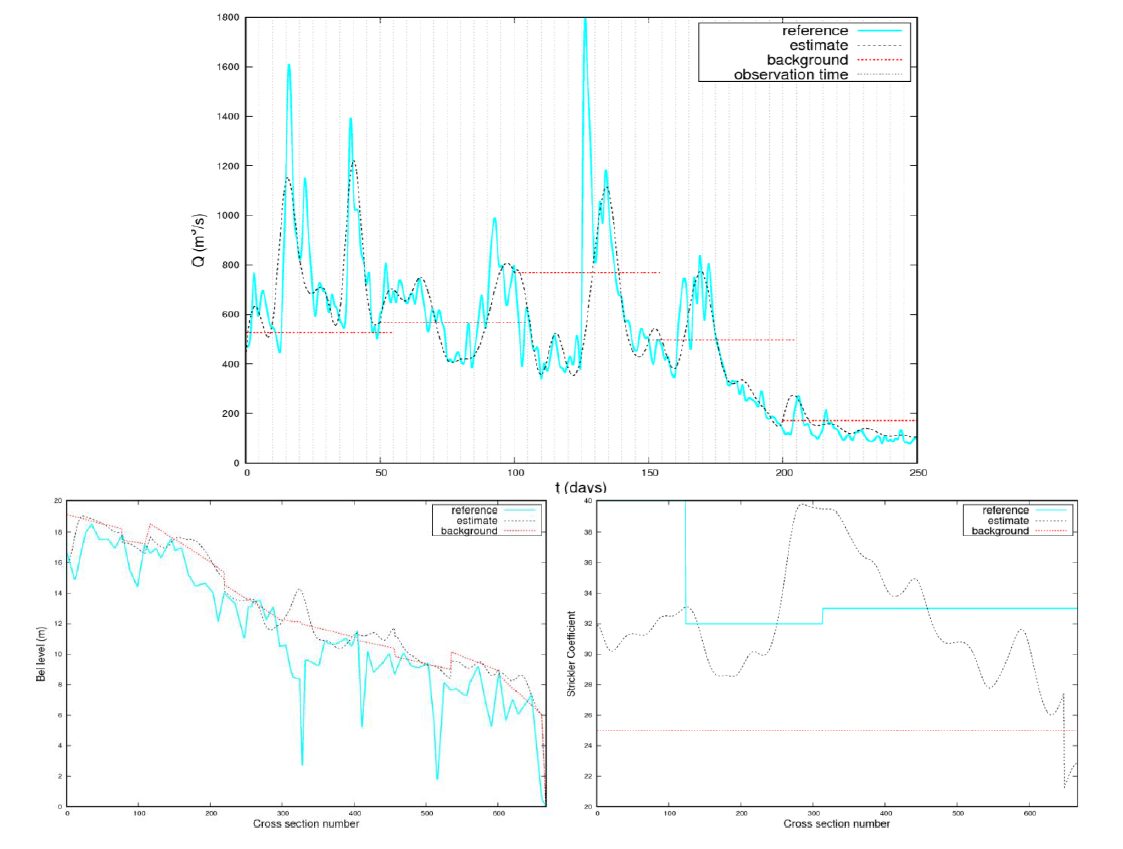## River Discharge Estimation Under Uncertainty from Synthetic SWOT-Type Observations Using Variational Data Assimilation

Contribution of the UMR-GEAU Investigation Team, IRSTEA Montpellier, France to the SWOT Mission
Hind Oubanas, Igor Gejadze, Pierre-Olivier Malaterre (Irstea - Montpellier, France)

### 1. Introduction & Objectives

The GHOSTE Team (Gestion Hydraulique, Optimisation et Supervision des Transferts d'Eau) is part of the UMR G-eau (Unité Mixte de Recherche sur la Gestion de l'Eau, de ses Acteurs et de ses Usages), located at Irstea, Montpellier, France. This Team has more than 30 years' experience in hydraulic modeling, software development (SIC2 software and some others), automatic control, dam management and data assimilation (Particle Filters, Kalman Filters, Variational methods) on open surface hydraulic systems such as Rivers and Irrigation Canals. The upcoming Surface Water and Ocean Topography (SWOT) mission is a challenging project and an opportunity to test and further develop algorithms using its scientific and technical resources.

Variational data assimilation has been applied for discharge estimation under uncertainties in river bathymetry and bed roughness using the hydraulic model . Synthetic WSE measurements, which emulate the spatial and temporal sampling of the SWOT mission, are assimilated for the Garonne River downstream reach. Similar tests have been carried out on the Po and Sacramento Rivers using the SWOT hydrology simulator developed at JPL. We investigate simultaneous estimation of discharge, river bathymetry and bed roughness in the framework of the extended control vector approach.

### 2.1 Hydraulic Model: 1.5D SIC2

SIC2 is a one-dimensional hydraulic model developed at Irstea-Montpellier (previously Cemagref) (http://sic.g-eau.net/). This software is dedicated to modeling the flow dynamics of rivers, irrigation canals and drainage networks. The model inputs, in its classical direct mode, include initial condition on discharge Q(x,t) and WSE Z(x,t), usually given by a steady flow solution, upstream and downstream boundary conditions on discharge Q(x,t0) and/or WSE Z(x,t0). A-priori information on the channel bed level Zb(x) along with the complete description of cross-sections of any shape, including the top width W(x) and roughness, given by the Strickler coefficient Cs(x), should be provided.

SIC2 solves the full 1D Saint-Venant equations for steady and unsteady open channel flow:

Where QL(x,t) refers to the channel lateral discharge, A(Z(x,t)) is the wetted cross-sectional area, represents the Sf=Q2/(CS2A2R4/3) friction term, R is the hydraulic radius, Ck is the lateral discharge momentum coefficient and v=Q/A is the mean velocity. The four-point implicit finite-difference method, Preissmann scheme, is used to find the numerical solution of these equations.

Data Assimilation methods have been widely used in environmental applications to find the best estimate of the 'control' variables. The control vector, denoted U, usually consists on the state variables and parameters which govern the behavior of the system (initial state, boundary conditions, model parameters, etc.). Most DA methods aim to combine the contributions from different sources of information (observations, a priori knowledge called the 'background', etc.), weighted by their respective approximate variance. In practice, the available observations Y and background Ub are corrupted by noise. Therefore, the observation error ξ0 and the background error ξb are introduced such that Y=Yt + ξ0 and Ub=Utb, where Yt and Ut assumed to be the 'true' values of Y and Ub, respectively. In this respect, the variational DA approach gives the best estimate of the control vector by minimizing a cost function. Under the Gaussian assumptions on the observation and the background error probability distribution, the classical formulation is given by a weighted quadratic cost function to be minimized, such that, U=arg minu J(U), for:

where R=E(ξ0 ξ0T) and B=E(ξbξbt) are the observation and the background error covariance matrices, respectively, and G:U->Y represents the nonlinear operator that maps the model variables into the observation space.

The stability of the minimization procedure can be achieved by introducing the change of variables U=Ub + B1/2W. We use the iterative regularization method, based on the semi-convergence of the solution and the following discrepancy principle J(W)≈X2(m,δ), where m and δ are the observation space dimension and the confidence level, respectively. The minimization problem becomes:

The minimization of the cost function is achieved by using iterative methods, such as quasi-Newton methods. For example, the limited-memory Broyden–Fletcher–Goldfarb–Shanno (LBFGS) update step has the form:

where, at the ith iteration, ßi is the descent step and Hi-1 is the approximated inverse of the Hessian. The gradient of the cost function at has the form:

where, G'(Ui) and (G'(Ui))* are respectively the tangent linear model and the adjoint model of the nonlinear operator G(Ui), given by the following Gâteaux derivative:

The tangent linear and adjoint models of SIC2 have been produced using the automatic differentiation tool TAPENADE developed by INRIA (France). For further information, the reader can refer to the related papers stated in section 5.

### 3. Experimental Design

The study area is a 50 km downstream reach of the Garonne River, France, flowing from Tonneins at the upstream to La Réole at the downstream. We focus on the flooding events that occurred during the year 2010, particularly during the period January-August, with a maximum discharge value of 1800 m3/s.

This study aims to assess the performance of the variational DA in discharge estimation under uncertainties, bringing to light the utility of the SWOT measurements. We investigate the estimation of the upstream discharge boundary condition Q(xup, t) (flood hydrograph), located at Tonneins. In this respect, the identical twin experiment framework has been considered. We use SIC2 simulation to obtain the synthetic SWOT measurements of WSE, spatially distributed each 10km, with an error standard deviation equal to 10cm.

The initial guess on discharge is taken as the mean annual value at Tonneins, being constant during the assimilation sub-window. The first guess for the subsequent sub-window is taken from the final time estimate of the previous sub-window. From the available observations (water level and width) and the Manning's equation under the rectangular assumption of the cross-sections shape, and uniform flow, the first guess on the bathymetry and the friction coefficient are generated. These priors are generated in the framework of the SWOT Discharge Algorithm Working Group experimental design ("The Pepsi Challenge").

### 4. Results and Discussion

The results of the simultaneous estimation of discharge, bed level and friction coefficient are illustrated in Figure 1 and Figure 2, for the following time frequencies: 1) 1-day, which is the time frequency used in the Pepsi Challenge, 2) 5-day, which is the expected SWOT time frequency in the mid-latitude.

Starting from non-informative first guess, discharge is successfully estimated, with rRMSE=4.6%, when the temporal frequency is comparable with the characteristic time of the dynamical system. For smaller frequencies, unobserved intervals may lead to inaccurate results, with rRMSE=24.1%, if no complementary information is provided (informative background, in-situ measurements). The uncertainty in the bathymetry and the friction has been explicitly treated via the simultaneous estimation of discharge, bed level and friction coefficient. The discharge is very well recovered during observation times, whereas the estimates of the bed level and the friction coefficient only help to achieve a better estimation quality for discharge. Therefore, these estimates may not be usable for subsequent experiments of data assimilation or for model calibration.Figure 1. Estimation of discharge under uncertainty in the bed level and Strickler coefficient using simplified rectangular bathymetry. Observation frequency: 1day.Figure 2. Estimation of discharge under uncertainty in the bed level and Strickler coefficient using simplified rectangular bathymetry. Observation frequency: 5day.

Similar tests and results have been obtained on the Po and Sacramento River using the SWOT simulator outputs. The corresponding results are under publication. The objective of the team is to further test and develop this algorithm, on various situations, such as the ones of the Pepsi and DA Pepsi Challenges.

### 5. Papers & Publications

Gejadze, I., P.-O. Malaterre (2017), Discharge estimation under uncertainty using variational methods with application to the full Saint-Venant hydraulic network model, International Journal for Numerical Methods in Fluids, 83(5), 405–430.

Gejadze, I. Y., P.-O. Malaterre (2016), Design of the control set in the framework of variational data assimilation, Journal of Computational Physics, 325, 358–379.

Oubanas, H., Gejadze, I., Malaterre, P.-O., Mercier, F. Estimation of river discharge from in-situ and remote sensing data, using variational data assimilation and a full Saint-Venant hydraulic model. 3rd Space for Hydrology Workshop, Frascati (Rome), Italy, 09/2015.

Oubanas, H., Gejadze, I., Malaterre, P.-O., Durand, M., Wui, R., Domeneghetti, A. Simultaneous estimation of inflow discharge, river bathymetry and friction from synthetic SWOT data using variational data assimilation. AGU Fall Meeting, San Francisco, California, USA, 12/2016.

#### Coming Papers

Oubanas, H., Gejadze, I., Malaterre, P.-O., Mercier, F. (2017), River discharge estimation from synthetic SWOT-type observations using variational data assimilation, Journal of Hydrology.

Oubanas, H., Gejadze, I., Malaterre, P.-O., Durand, M., Wei, R., Frasson, R., Domeneghetti, A. (2017), SWOT mission for discharge estimation under uncertainties using variational data assimilation, Water Resources Research.

### Acknowledgements

The team thanks Franck Mercier (CLS) for its implication in the project, Nicole Goutal (EDF) and Sophie Ricci (Cerfacs) for providing the downstream Garonne data and the constructive discussions on hydraulic modeling, and Michael Durand (OSU) for the Pepsi-Challenge a-priori information. Hind Oubanas' PhD grant is funded by Irstea and CLS.